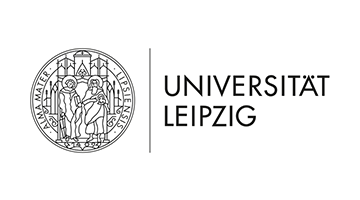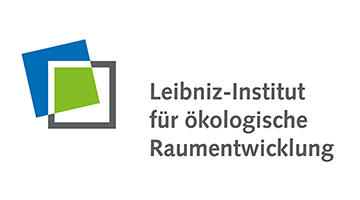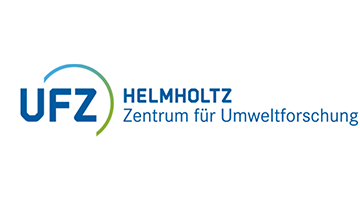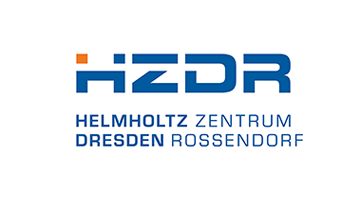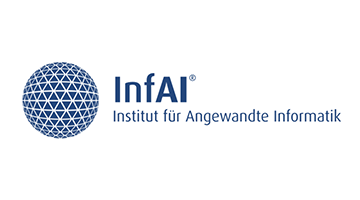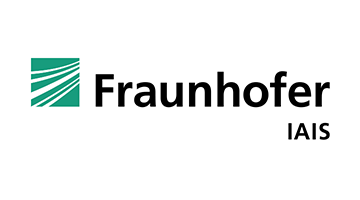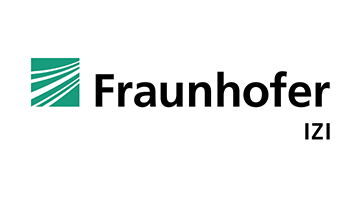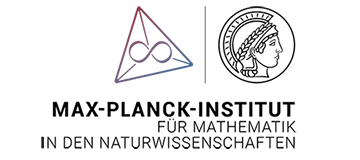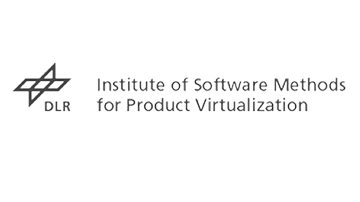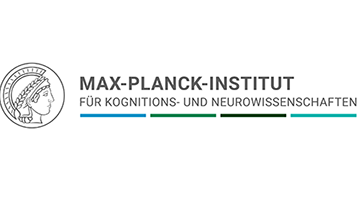# Image Recognition with asanAI

Image Recognition is one of many functionalities of asanAI. For images, the detection process is a bit more complicated than just dense layers. One technique has been very successful. It’s called convolution. A convolution is a matrix operation.

### How Image Recognition with asanAI works

Suppose we have the following image matrix, which encodes an image.

We then also have another matrix of a smaller size, which is called a filter. The filter could look like this:

We then slide this matrix over the image and multiply the submatrices from the image with the filter.

This is the first submatrix we consider:

We put this into the output matrix. We then go next to next 2×2 (filter size) submatrix. This is:

Afterwards, we proceed with the same filter. We continue this through the whole image. These filters can be detect some structures in the image. Please, be aware of the filter size and how the output of a convolution is generated. Conv2d Layers work like this on 2d image data. In a conv2d layer, you may have multiple filters. Each filter may detect a different type of structure. The first layer may detect very simple geometric features, the second layer may detect features that are built up from features detected in the previous layer and so on.

After every layer, there can be activation functions again, that can, for example, „squeeze“ the values between 0 and 1. The more layers and filters you have, the more complex stuff your network can detect. If you want classification, then, after having a bunch of convolution layers, you need to flatten. That means: from any tensors, a vector with it’s values will be created.

Example:

After this, you can use Dense Layers to classify the resulting data. An example structure would be the Network, 3 Conv2d Layers followed by a flatten and 2 Dense Layers. The resulting output vector contains 10 values, each being a probability (given by SoftMax activation) in percent of how likely a number image is a certain number.

### Train your own image classification network

Chose Problem type: Image Classification. For simple problems, you can try the Digit Classifier Network first. Chose X&Y-Source: Own and Data Type: Image. It is recommended that you resize your images before you put them into asanAI, because memory is scarce. You can add images there and click Start Training. You can, in the predict tab, see what your prediction looks like. If the model does not detect what you want it to detect, you can try adding more conv2d layers or more filters at the beginning of the network or dense layers at the end. You can, after training, click on Settings and Visualize Layer. This calculates the input that maximally excites a single neuron/filter. If this is random noise, the filter has not learned anything. If there are any patterns, these patterns are what the filter looks for when predicting.